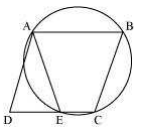Guru

# ABCD is a parallelogram. The circle through A, B and C intersect CD (produced if necessary) at E. Prove that AE, = AD. Q.6

• 0

Give me the best and simple way for solving the question of class 9th of Circles chapter of math of question no.6 how i solve this question in easy and simple way because it is very important type of question ABCD is a parallelogram. The circle through A, B and C intersect CD (produced if necessary) at E. Prove that AE, = AD.

Share

1.Here, ABCE is a cyclic quadrilateral. In a cyclic quadrilateral, the sum of the opposite angles is 180°.

So, ∠AEC+∠CBA = 180°

As ∠AEC and ∠AED are linear pair,

∠AEC+∠AED = 180°

Or, ∠AED = ∠CBA … (1)

We know in a parallelogram; opposite angles are equal.

So, ∠ADE = ∠CBA … (2)

Now, from equations (1) and (2) we get,